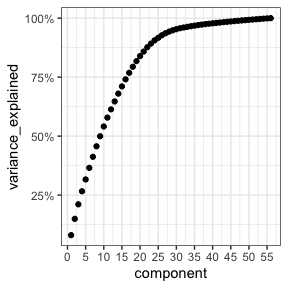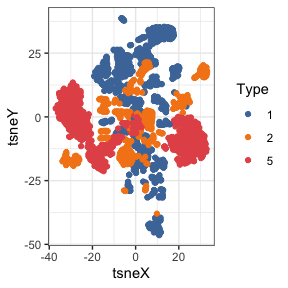# Using liminal to understand high dimensional parameter space

This example is modified from the examples tours described in . Here we use a tour to explore principal components space and any non-linear structure and clusters via t-SNE.

## Setting up the data

Data were obtained from CT14HERA2 parton distribution function fits as used in . There are 28 directions in the parameter space of parton distribution function fit, each point in the variables labelled X1-X56 indicate moving +- 1 standard deviation from the ‘best’ (maximum likelihood estimate) fit of the function. Each observation has all predictions of the corresponding measurement from an experiment. (see table 3 in that paper for more explicit details).

The remaining columns are:

• InFit: A flag indicating whether an observation entered the fit of CT14HERA2 parton distribution function
• Type: First number of ID
• ID: contains the identifier of experiment, 1XX/2XX/5XX corresponds to Deep Inelastic Scattering (DIS) / Vector Boson Production (VBP) / Strong Interaction (JET). Every ID points to an experimental paper.
• pt: the per experiment observational id
• x,mu: the kinematics of a parton. x is the parton momentum fraction, and mu is the factorisation scale.

First, we take the load the data as a data.frame:

library(liminal)
data(pdfsense)

## Linear embeddings and the tour

First we can estimate all nrow(pdfsense) principal components using on the parton distribution fits:

pcs  <- prcomp(pdfsense[, 7:ncol(pdfsense)])

Using this data structure, we can produce a screeplot:

res <- data.frame(
component = 1:56,
variance_explained = cumsum(pcs$sdev / sum(pcs$sdev))
)

ggplot(res, aes(x = component, y = variance_explained)) +
geom_point() +
scale_x_continuous(
breaks = seq(0, 60, by = 5)
) +
scale_y_continuous(
labels = function(x) paste0(100*x, "%")
)Approximately 70% of the variance in the pdf fits are explained by the first 15 principal components.

Next we augment our original data with the principal components:

pdfsense <- dplyr::bind_cols(
pdfsense,
as.data.frame(pcs$x) ) pdfsense$Type <- factor(pdfsense$Type) We can view a simple tour vialimn_tour() and color points by their experimental group limn_tour(pdfsense, PC1:PC6, Type) ## Non-Linear embeddings Now we can set up a non-linear embedding via t-SNE, here we embed all 56 principal components. set.seed(3099) start <- clamp_sd(as.matrix(dplyr::select(pdfsense, PC1, PC2)), sd = 1e-4) tsne <- Rtsne::Rtsne( dplyr::select(pdfsense, PC1:PC56), pca = FALSE, normalize = TRUE, perplexity = 50, exaggeration_factor = nrow(pdfsense) / 100, Y_init = start ) Once we have run t-SNE we tidy it into a data.frame, to perform a linked tour. tsne_embedding <- as.data.frame(tsne$Y)
tsne_embedding <- dplyr::rename(tsne_embedding, tsneX = V1, tsneY = V2)
tsne_embedding$Type <- pdfsense$Type

We can view the clusters using a static scatter plot:

ggplot(tsne_embedding,
aes(x = tsneX, y = tsneY, color = Type)) +
geom_point() +
scale_color_manual(values = limn_pal_tableau10())We can link a tour view next to the embedding to give us a clear picture of the clustering:

limn_tour_link(
tour_data = pdfsense,
embed_data = tsne_embedding,
cols = PC1:PC6,
color = Type
)